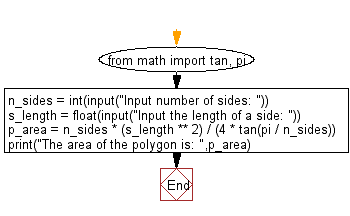﻿ Python Math: Calculate the area of a regular polygon - w3resource# Python Math: Calculate the area of a regular polygon

## Python Math: Exercise-28 with Solution

Write a python program to calculate the area of a regular polygon.

Sample Solution:-

Python Code:

``````from math import tan, pi
n_sides = int(input("Input number of sides: "))
s_length = float(input("Input the length of a side: "))
p_area = n_sides * (s_length ** 2) / (4 * tan(pi / n_sides))
print("The area of the polygon is: ",p_area)
```
```

Sample Output:

```Input number of sides: 4
Input the length of a side: 20
The area of the polygon is:  400.00000000000006
```

Pictorial Presentation:Flowchart:## Visualize Python code execution:

The following tool visualize what the computer is doing step-by-step as it executes the said program:

Python Code Editor:

Have another way to solve this solution? Contribute your code (and comments) through Disqus.

What is the difficulty level of this exercise?

Test your Programming skills with w3resource's quiz.

﻿

```>>> students = [{'name': 'John', 'score': 98}, {'name': 'Mike', 'score': 94}, {'name': 'Jennifer', 'score': 99}]